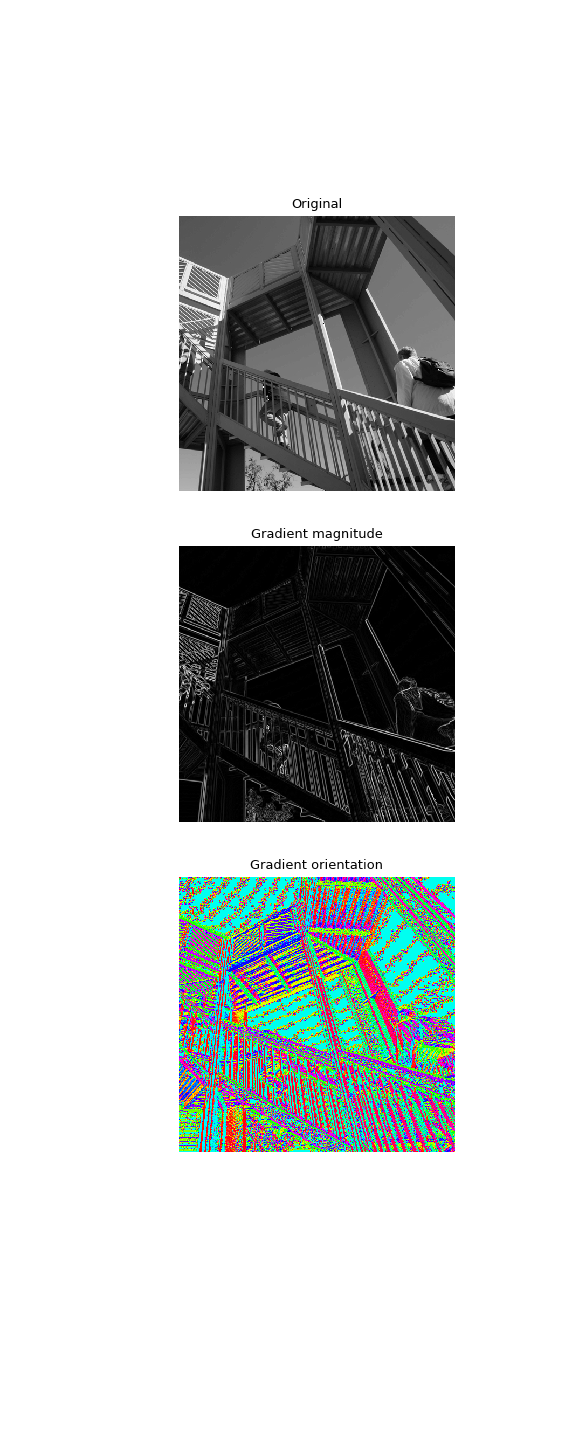# scipy.signal.convolve2d¶

scipy.signal.convolve2d(in1, in2, mode='full', boundary='fill', fillvalue=0)[source]

Convolve two 2-dimensional arrays.

Convolve in1 and in2 with output size determined by mode, and boundary conditions determined by boundary and fillvalue.

Parameters: in1 : array_like First input. in2 : array_like Second input. Should have the same number of dimensions as in1. If operating in ‘valid’ mode, either in1 or in2 must be at least as large as the other in every dimension. mode : str {‘full’, ‘valid’, ‘same’}, optional A string indicating the size of the output: full The output is the full discrete linear convolution of the inputs. (Default) valid The output consists only of those elements that do not rely on the zero-padding. same The output is the same size as in1, centered with respect to the ‘full’ output. boundary : str {‘fill’, ‘wrap’, ‘symm’}, optional A flag indicating how to handle boundaries: fill pad input arrays with fillvalue. (default) wrap circular boundary conditions. symm symmetrical boundary conditions. fillvalue : scalar, optional Value to fill pad input arrays with. Default is 0. out : ndarray A 2-dimensional array containing a subset of the discrete linear convolution of in1 with in2.

Examples

Compute the gradient of an image by 2D convolution with a complex Scharr operator. (Horizontal operator is real, vertical is imaginary.) Use symmetric boundary condition to avoid creating edges at the image boundaries.

>>> from scipy import signal
>>> from scipy import misc
>>> ascent = misc.ascent()
>>> scharr = np.array([[ -3-3j, 0-10j,  +3 -3j],
...                    [-10+0j, 0+ 0j, +10 +0j],
...                    [ -3+3j, 0+10j,  +3 +3j]]) # Gx + j*Gy
>>> grad = signal.convolve2d(ascent, scharr, boundary='symm', mode='same')

>>> import matplotlib.pyplot as plt
>>> fig, (ax_orig, ax_mag, ax_ang) = plt.subplots(3, 1, figsize=(6, 15))
>>> ax_orig.imshow(ascent, cmap='gray')
>>> ax_orig.set_title('Original')
>>> ax_orig.set_axis_off()